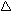# Solar Science Model Explains How It Works

### 1994 Scoping Analysis SolarAtticTM Solar Pool Heater

From the handwritten notes of the 11/29/93 "scoping analysis" performed by
Professor Ephraim M. Sparrow, University of Minnesota, Minneapolis, MN.

Heat Supply To Attic Air :

1. Direct solar. Use 250 Btu/hr ft2 incident normal to the sun's rays. The angle of incidence varies throughout a given day and also depends on latitude and on the season. Use a factor of 0.7 to account for the non-normal incidence. As a best case, assume that all the incident solar is absorbed (i.e., d s= 1). If a 1500 ft 2 roof is everywhere incident by direct solar, then

250 x 0.7 x 1500 = 262,500 Btu/hr.

This would be valid for an unshaded, low-pitch roof. Perhaps a more typical roof
would be half shadowed. For such a roof, the absorbed solar would be @ 130,000
Btu/hr.

1. Scattered Solar.

Use 30 Btu/hr - ft 2 as a typical value. For total absorption on a 1500 ft2 roof

30 x 1500 = 45,000

1. Total solar. Range:

175,000 - 307,500

Heat Losses From Attic Roof:

1. Convection losses. Assume roof outside surface temperature is 115 degrees F and that the air ambient is 85 degrees F.
2. Convective heat loss equation:

Q conv =T/R, R= 1/hA

where R is the thermal resistance without wind,

h @ 0.7 Btu/hr-ft2 -° F.

So that

 (115 - 85) Q conv = ____1____ = 31,500 Btu/hr (0.7) (1500)

For moderate wind, use h @ 2 Btu/hr-ft 2 -° F

Then,

Q loss = (115 - 85)/ (1/(2)(1500))

Q loss = 90,000 Btu/hr

1. Radiation losses. If the diffuse sky radiation is characterized by T sky, then,

Q loss = E s A (T4 -T sky4 )

This equation is for infrared radiation. The emissivity E is about 0.85 for both
black and white roof surfaces;

s = 0.1712 x 10 -8 Btu/ hr-ft2 -° R4 .

Both T and T sky are in degrees Rankine, ° R = ° F+ 460. Then,

Q loss = (0.85) (0.1712 x 10 -8 ) (1500) (575 4 - 4604 )

Q loss = 141,000 Btu/hr.

Heat Balance:

Max Q loss = 90,000 + 141,000

= 231,000 Btu/hr

Min Q loss = 31,500 + 141,000

= 172,500 Btu/hr

Range of losses: 172,500 - 231,000 Btu/hr

Range of solar absorbed: ( a s = 1)

175,000 - 307,500

Conclusion: There are clearly many cases where the system will work (i.e. provide = 90,000 Btu/hr), but the combination of a half or more shadowed roof and moderate wind will unable the [pool] water heating to be accomplished.